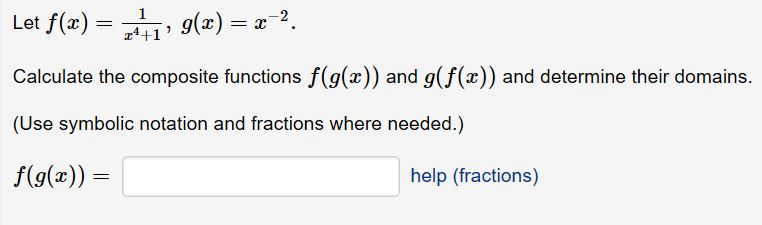# Let f(z) = , g(x)-z-2. Calculate the composite functions f(g(x)) and g(f(x)) and determine their domains. (Use symbolic notation and fractions where needed.) f(g(x) a4+1 help (fractions)

Question

Help.help_outlineImage TranscriptioncloseLet f(z) = , g(x)-z-2. Calculate the composite functions f(g(x)) and g(f(x)) and determine their domains. (Use symbolic notation and fractions where needed.) f(g(x) a4+1 help (fractions) fullscreen

### Want to see this answer and more?

Experts are waiting 24/7 to provide step-by-step solutions in as fast as 30 minutes!*

*Response times vary by subject and question complexity. Median response time is 34 minutes and may be longer for new subjects.
Tagged in
MathCalculus

### Functions